• 本文主要讲解随机事件的概念，事件间的关系和运算；随机事件的概率定义，概率的性质
在现实社会和工程领域、科学实验中，存在着各种各样的现象，有一类现象被称为确定性现象；另一类现象被称为不确定性现象，这些不确定性现象又可以归为两类：随机性现象和模糊性现象。

随机现象：在一定条件下可能发生种种不同结果的现象称为随机现象。；比如投掷一枚硬币，出现的结果可能是正面，也可能是反面。
模糊现象：模糊性指客观事物的差异在中介过渡中所呈现的“亦此亦彼”性，造成事物的结果不清晰。例如我们说气候冷热，体型胖瘦等概念，都具有含义不确切，边界不清晰等模糊性，这种有模糊性的现象叫模糊现象。
概率论和数理统计是研究和揭示随机现象统计规律性的一门数学学科。

本文主要讲解随机事件的概念，事件间的关系和运算；随机事件的概率定义，概率的性质。

1 随机事件

1,1概念

试验E的所有可能结果组成的集合为E的样本空间，记为S。样本空间的元素即试验E的每个可能结果称为样本点，记为e。由S中的一些样本点组成的集合称为随机试验E的随机事件，简称事件。

必然事件：在每次试验中必然发生的事件，称为E的必然事件。
不可能事件：在每次试验都不可能发生的事件，称为E的不可能事件。
1.2事件间的关系和运算

事件的包含与相等：

设有两个事件A和B，若事件A发生时B必发生，则称事件B包含事件A，或者事件A包含于事件B，记为A⊂B，也称A是B的子事件。

若 A⊂B并且B⊂A，则称A，B两事件相等，记为A=B。

事件的和与积：

事件A与事件B中至少有一个事件发生，称为A，B的和事件，记为A∪B。

事件A与事件B同时发生，称为A，B的积事件，记为A∩B，简记为AB。

互不相容事件与对立事件：

若事件A与B不可能同时发生，即AB=∅，则称A与B为互不相容事件，也称为互斥事件。

若事件B={A不发生}，则称B为A的对立事件，记为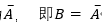事件的差：

事件A发生，事件B不发生，称为A与B的差事件，记为A-B。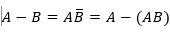事件关系的几何表示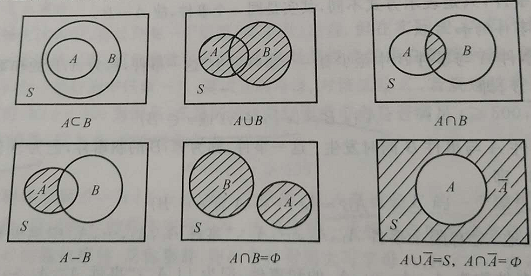事件的运算规律

交换律：A∪B=B∪A, AB=BA

结合律：A∪BUC=A∪(B∪C), (AB)C = A(BC)

分配率：A∪BC=(AC)∪(BC), AB∪C=(A∪C)(B∪C)

对偶率：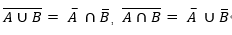对于事件A，B， 若A⊂B，则有A∪B=B, A∩B=A

2随机事件的概率

2.1古典概型

定义

若随机试验具有以下两个特点：

试验的可能结果只有有限个，即样本空间只包含有限个样本点
每个试验结果在一次试验中出现的可能性是相同的
则称此类随机试验的概率模型为古典概型（或等可能概型）。

事件A的古典概率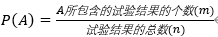古典概率有以下性质：

对任意事件A，0≤P(A)≤1
对于必然事件S，不可能事件∅，有P(S)=1, P(∅)=0
对于互斥事件A,B， 即AB=∅, 有 P(A∪B)=P(A)+P(B)
2.2 概率定义

随机事件有一个重要特性：频率稳定性，即当试验次数充分大时，事件A出现的频率总在[0,1]区间的每个确定的常数p附近摆动。

概率的统计定义

设有试验E，若当试验的重复次数n充分大时，事件A发生的频率稳定的在某常数p附近摆动，则称常数p为事件A的概率，记为P(A)=p

概率的公理化定义

设随机试验E的样本空间是S，对E的每一个事件A，赋予一个实数，记为P(A)，如果函数集合P(.),满足下列条件：

非负性：对于任意事件A，有0≤P(A)≤1
规范性：对于必然事件S，有P(S)=1
可列可加性：若事件A1, A2, …, An,… 两两互斥，即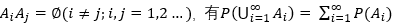则称P(.)为概率函数，简称概率，函数值P(A)称为事件A的概率。

2.3概率的性质

概率具有以下基本性质：

（1）对于任意事件A，有0≤P(A)≤1

（2）P(S)=1, P(∅)=0

（3）若事件A1, A2, …, An,… 两两互斥， 即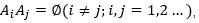则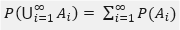（4）对任意事件A，有P(A)=1-P(A)

(5)  设A, B为两个随机事件，且 A⊃B,则P(A-B)=P(A)-P(B), P(A)>=P(B)

(6)   设A,B为任意两个随机事件，则

P(A∪B)=P(A)+P(B)-P(AB)


展开全文• 概率的公理化定义及概率的性质
§1.3概率的公理化定义及概率的性质 $\color{blue}{\S 1.3 概率的公理化定义及概率的性质}$

定义:设E是一个随机试验,Ω是基本空间,对E的每一个事件A,如果存在着一个实数(记作P(A)),它满足以下三个条件:(i)P(A)≥0,(非负性);(ii)P(Ω)=1,(规范性);(iii)对于两两互不相容的事件,A 1 ,A 2 ,⋯,有P(A 1 ∪A 2 ∪⋯)=P(A 1 )+P(A 2 )+⋯,(P(∑ i=1 ∞ A i )=∑ i=1 ∞ P(A i ))(可列可加性)则称P(A)为事件A的概率. ${\color{blue}{定义:设E是一个随机试验, \Omega是基本空间, \\ 对E的每一个事件A,如果存在着一个实数(记作P(A)),\\ 它满足以下三个条件: \\ (i) P(A) \geq 0,(非负性); \\ (ii) P(\Omega) = 1,(规范性); \\ (iii) 对于两两互不相容的事件, A_1, A_2, \cdots, 有\\ \qquad P(A_1 \cup A_2 \cup \cdots) = P(A_1) + P(A_2) + \cdots , \\ \qquad \Big( P(\sum \limits _ {i = 1} ^ {\infty} A_i) = \sum \limits _ {i = 1} ^ {\infty} P(A_i) \Big) \quad (可列可加性) \\ 则称P(A)为事件A的概率. }}$

性质1.P(ϕ)=0. $性质1.P(\phi) = 0.$
证:由ϕ=ϕ∪ϕ∪⋯,再根据可列可加性,有P(ϕ)=P(ϕ)+P(ϕ)+P(ϕ)+⋯,又因为P(ϕ)≥0,所以必有P(ϕ)=0. $证:由\phi = \phi \cup \phi \cup \cdots, 再根据可列可加性,有 \\ \qquad P(\phi) = P(\phi) + P(\phi) + P(\phi) + \cdots, \\ 又因为 P(\phi) \geq 0,所以必有 P(\phi) = 0 .$

性质2.设A 1 ,A 2 ,⋯,A n 为n个互不相容的事件,则有P(⋃ i=1 n A i )=∑ i=1 n P(A i )(P(∑ i=1 n A i )=∑ i=1 n P(A i ))(有限可加性). $性质2.设A_1, A_2, \cdots, A_n为n个互不相容的事件,则有 \\ P(\bigcup \limits _ {i = 1}^{n} A_i) = \sum \limits _ {i = 1} ^ {n} P(A_i) \\ \Big( P(\sum \limits _ {i = 1} ^ {n} A_i ) = \sum \limits _ {i =1} ^ {n} P(A_i) \Big) \quad (有限可加性).$
证:令A n+1 =A n+2 =⋯=ϕ,则A 1 ,A 2 ,⋯,A n ,A n+1 ,A n+2 ,⋯为两两互不相容的事件,由可列可加性及性质1,有P(⋃ i=1 n A i )=P(⋃ i=1 ∞ A i )=∑ i=1 ∞ P(A i )=∑ i=1 n P(A i ). $证:令 A_{n+1} = A_{n+2} = \cdots = \phi, \\ 则A_1, A_2, \cdots, A_n, A_{n + 1}, A_{n+2}, \cdots 为\\ 两两互不相容的事件,由可列可加性及性质1,有 \\ P(\bigcup \limits _ {i = 1} ^ {n} A_i) = P(\bigcup \limits _ {i = 1} ^ {\infty} A_i) = \sum \limits _ {i = 1} ^ {\infty} P(A_i) = \sum \limits _{i = 1} ^ {n} P(A_i) .$

性质3.对任意事件A,有P(A)=1−P(A ¯ )或P(A ¯ )=1−P(A).或P(A)+P(A ¯ )=1. $性质3.对任意事件A,有P(A) = 1 - P( \bar A) 或 P( \bar A) = 1 - P(A). \\\\ 或P(A) + P(\bar A) = 1.$
证:由于A与A ¯ 互不相容,且A∪A ¯ =Ω,所以有1=P(Ω)=P(A∪A ¯ )=P(A)+P(A ¯ ),即P(A)=1−P(A ¯ )或P(A ¯ )=1−P(A). $证:由于A与\bar A互不相容, 且 A \cup \bar A = \Omega, 所以有 \\ 1 = P(\Omega) = P(A \cup \bar A) = P(A) + P(\bar A), \\ 即 P(A) = 1 - P(\bar A) 或 P(\bar A) = 1 - P(A).$

性质4.若A⊂B,则有P(B−A)=P(B)−P(A),且P(A)≤P(B). $性质4.若 A \subset B,则有P(B - A) = P(B) - P(A),且P(A) \leq P(B).$
证:由于A⊂B,则有B=A∪(B−A),且A∩(B−A)=ϕ.于是有P(B)=P(A∪(B−A))=P(A)+P(B−A),即P(B−A)=P(B)−P(A),再由P(B−A)≥0,∴且P(A)≤P(B). $证:由于A \subset B,则有B = A \cup (B - A),且 A \cap (B - A) = \phi. \\ 于是有P(B) = P(A \cup (B - A)) = P(A) + P(B - A), \\ 即P(B - A) = P(B) - P(A), \\ 再由P(B - A) \geq 0,\therefore 且P(A) \leq P(B).$

性质5.对于任一事件A,有P(A)≤1 $性质5. 对于任一事件A,有 P(A) \leq 1$
证:因A⊂Ω,则有P(A)≤P(Ω)=1. $证: 因 A \subset \Omega, 则有 P(A) \leq P(\Omega) = 1.$

性质6.对任意两个事件A与B,有P(A∪B)=P(A)+P(B)−P(AB).(加法公式) $性质6.对任意两个事件A与B,有P(A \cup B) = P(A) + P(B) - P(AB). \quad (加法公式)$
证:A∪B=A∪(B−AB),A(B−AB)=ϕ,又AB⊂B,因此有P(A∪B)=P(A∪(B−AB))=P(A)+P(B−AB)=P(A)+P(B)−P(AB) $证: A \cup B = A \cup (B - AB) , A(B - AB) = \phi, \\ 又 AB \subset B,因此有\\ P(A \cup B) = P(A \cup (B - AB)) = P(A) + P(B - AB) \\ = P(A) + P(B) - P(AB)$

同时由P(AB)≥0有P(A∪B)≤P(A)+P(B) $同时由P(AB) \geq 0 有 P(A \cup B) \leq P(A) + P(B)$

推论:对任意三个事件A,B,C有P(A∪B∪C)=P(A)+P(B)+P(C)−P(AB)−P(AC)−P(BC)+P(ABC).(多除少补原理) $推论:对任意三个事件A,B,C有 \\ P(A \cup B \cup C) = P(A) + P(B) + P(C) - P(AB) - P(AC) - P(BC) + P(ABC). \\ (多除少补原理)$

例1.设P(A)=14 ,P(B)=13 ,A⊂B,求P(A ¯ ),P(B−A),P(A−B). $例1.设P(A) = \dfrac{1}{4}, P(B) = \dfrac{1}{3}, A \subset B, \\ 求P( \bar A), P(B-A), P(A-B).$
解:P(A ¯ )=1−P(A)=1−14 =34 .P(B−A)=P(B)−P(A)=13 −14 =112 .P(A−B)=P(ϕ)=0. $解:P(\bar A) = 1 - P(A) = 1 - \dfrac{1}{4} = \dfrac{3}{4}. \\ P(B - A) = P(B) - P(A) = \dfrac{1}{3} - \dfrac{1}{4} = \dfrac{1}{12}. \\ P(A - B) = P(\phi) = 0.$

例2.设P(A)=P(B)=P(C)=13 ,P(AB)=P(AC)=18 ,P(BC)=0,求P(A ¯ ),P(B−A),P(B∪C),P(A∪B∪C). $例2.设P(A) = P(B) = P(C) = \dfrac{1}{3}, P(AB) = P(AC) = \dfrac{1}{8}, \\ P(BC) = 0, 求P(\bar A), P(B - A), P(B \cup C), P(A \cup B \cup C).$
解:P(A ¯ )=1−P(A)=1−13 =23 P(B−A)=P(B−AB)=P(B)−P(AB)=13 −18 =524 P(B∪C)=P(B)+P(C)−P(BC)=13 +13 −0=23 由于BC⊃ABC,有0≤P(ABC)≤P(BC)=0,于是P(ABC)=0P(A∪B∪C)=P(A)+P(B)+P(C)−P(AB)−P(AC)−P(BC)+P(ABC)=34  $解:P(\bar A) = 1 - P(A) = 1 - \dfrac{1}{3} = \dfrac{2}{3} \\ P(B - A) = P(B - AB) = P(B) - P(AB) = \dfrac{1}{3} - \dfrac{1}{8} = \dfrac{5}{24} \\ P(B \cup C) = P(B) + P(C) - P(BC) = \dfrac{1}{3} + \dfrac{1}{3} - 0 = \dfrac{2}{3} \\ 由于BC \supset ABC, 有0 \leq P(ABC) \leq P(BC) = 0, 于是P(ABC) = 0 \\ P(A \cup B \cup C) = P(A) + P(B) + P(C) \\ - P(AB) - P(AC) - P(BC) + P(ABC) \\ = \dfrac{3}{4}$
展开全文• 概率的性质 1.P(φ) = 0（不可能事件的概率为0） 必然事件的概率为1，则概率为1的事件不一定是必然事件。 概率等于1的事件在某一次试验中不一定发生。 概率为0的事件不一定是不可能事件。（终点的概率为0，但不是不...
置项： 写好博客，参照here 或者复制打开下面链接

https://blog.csdn.net/cungudafa/article/details/84658703?depth_1-utm_source=distribute.pc_relevant.none-task&utm_source=distribute.pc_relevant.none-task

置项：使用LaTeX数学公式，参照here或者复制打开下面链接

https://katex.org/docs/supported.html

《概率论》概率的性质
文章目录~《概率论》~概率的性质1.P(φ) = 0（不可能事件的概率为0）2.有限可加性：3.对立事件的概率4.单调性5.概率的加法公式例题
1.P(φ) = 0（不可能事件的概率为0）
必然事件的概率为1，但概率为1的事件不一定是必然事件。
概率等于1的事件在某一次试验中不一定发生。
概率为0的事件不一定是不可能事件。（终点的概率为0，但不是不可能事件）。
2.有限可加性：
若事件A1,A2,…,An两两互斥，则有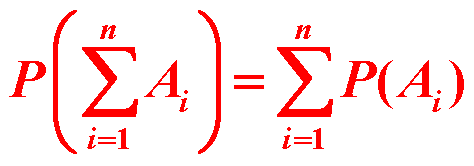3.对立事件的概率4.单调性
A,B为任意两个事件，且 $A \subset B$    ，则有5.概率的加法公式
A,B为任意两个事件，则有
P(A+B)=P(A)+P(B)-P(AB)
P(A+B+C)=P(A)+P(B)+P(\C)-P(AB)-P(AC)-P(BC)
推广：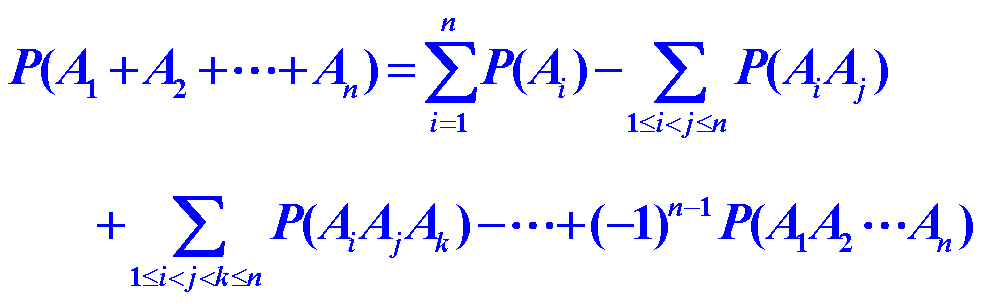例题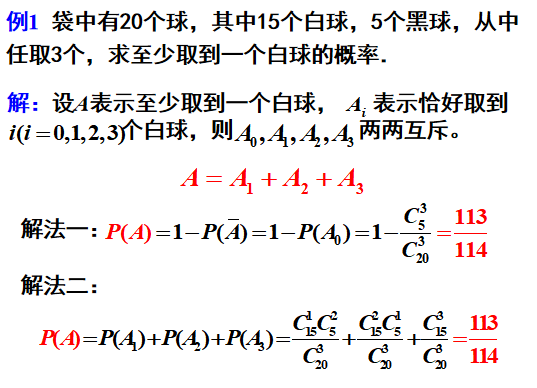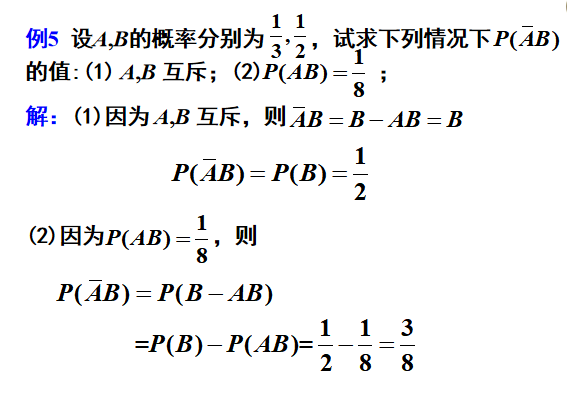展开全文• 概率的性质 （大写字母均代表事件） 概率的加法性质的公式 概率的连续性 定义 条件概率、乘法公式 定义 举个例子 重要公式 事件的独立性 独立性，指的是两个事件相互不产生影响。 定义 在这里需要注意的是...
参考美丽的小姐姐的笔记哦下面附上链接
https://zhuanlan.zhihu.com/p/42859784
概率的性质
（大写字母均代表事件）
概率的加法性质的公式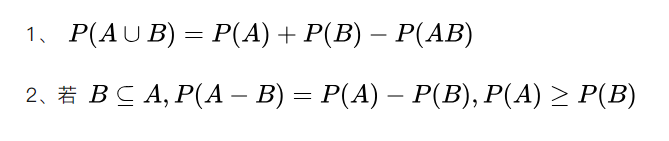概率的连续性
定义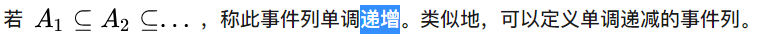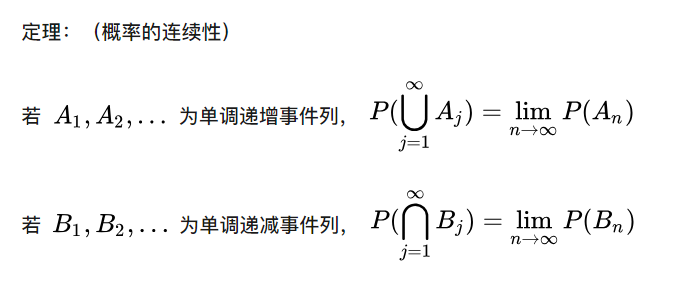条件概率、乘法公式
定义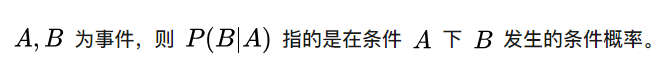举个例子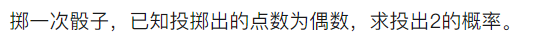重要公式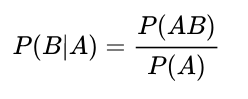事件的独立性
独立性，指的是两个事件相互不产生影响。
定义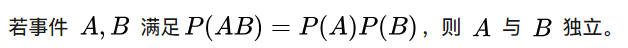在这里需要注意的是事件多于两个的情况。
相互独立定义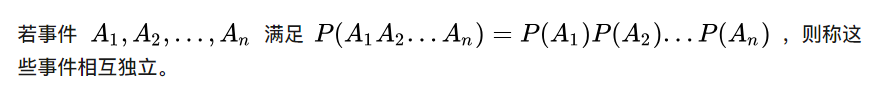相互独立则必定两两独立，但是反之不成立。
证明一下反之不成立的例子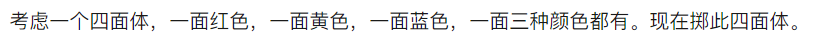解：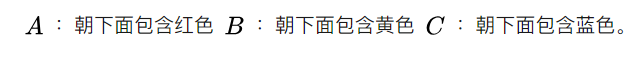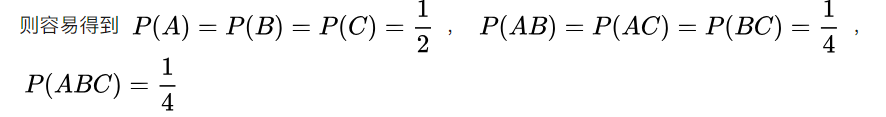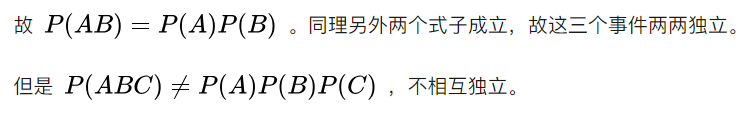（Bayes公式）贝叶斯公式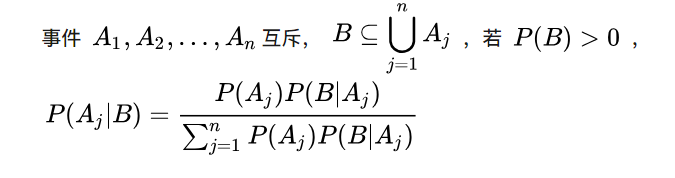例子解：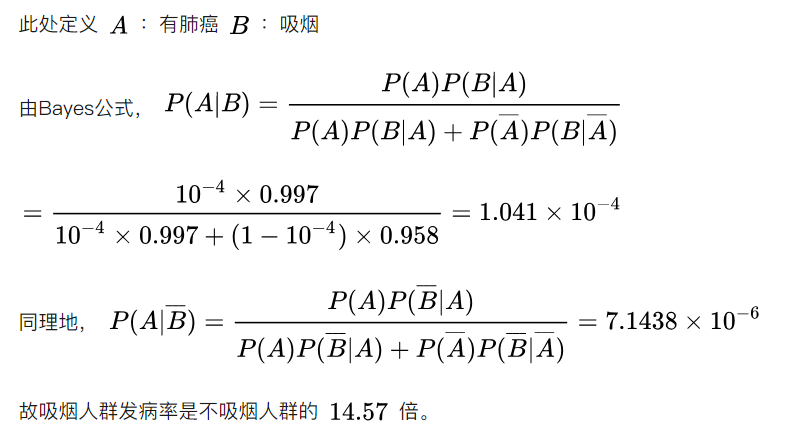展开全文• 本题就是考概率的性质：一共有四条：什么是概率来？？？详见扩展： 在记忆时，不要再受对立，互斥，独立这些概念的影响，对于任何情况，这些性质都是可以成立的。本题考到了性质2，互不相容（也就是互斥），对应...
• 文章目录随机事件的概率例题：附加： 随机事件的概率 概率 刻画随机事件发生可能性大小数量指标，是一个客观存在。 古典概型：--------常规题（不考难题） 特点： （1）试验所有可能出现基本事件只有...
• 概率的连续性如下定义：我们可以用韦恩图把他们表示出来，便于理解：图1 对应性质（1）图2 对应性质（2）从图1中我们可以看出，集合单调不增，打个比方，此集合会越来越小，那么称集合上连续（从上方逼近），极限为...
• 条件概率满足概率的所有性质，如： p(φ|B)=0, p(Ω|B)=1 P(AB)与P(A|B)的区别与联系： 联系： 两个都是在A,B发生情况下计算概率。 区别： (1) P(AB) 表示A,B同时发生的概率；P(A|B) 表示在B发生条件下A发生的...
• 使用LaTeX数学公式，参照here或者复制打开下面链接 https://katex.org/docs/supported.html 《概率论》概率的性质随机事件 文章目录 ~《概率论》~概率的性质随机事件 1.随机现象 2.随机试验 3.样本空间 4.随机事件与...
• 今天的主要内容为概率的一些基本公理以及它的证明和应用。慢慢的来，每天进步一丢丢。 概率三公理（Axioms of Probability） 公理是大家公认的定理，不需要证明，也无法证明。很多的学科的发展都是建立在一些...
• 最小二乘一次完成算法在具体实际使用过程中,不仅占用内存量大,还不适合在线...为了减少计算量,减少数据在计算机所占存储量,采用系统辨识里递推最小二乘法对一个实例进行公式推导,并进行了仿真和概率性质分析。
• 概率模型检查。 在Python中利用Storm功能。 上面库有其自己依赖性，需要首先解决。 安装 使用该工具简便方法是从docker镜像生成一个容器，该包含所有依赖项和预安装脚本。 入门 脚本需要参数如下：...Python
•研究论文
• 3. 古典概率Classical Probability 频率概率： 古典概型： 这个例子，n区别就是指定和没有指定。 后面365*364******* 意思是每个人生日都不一样，就是一个排列。题目要求至少两人，它对立面就是...
• 20世纪90年代C.Tricot给出了Hausdorff中心维数与Hausdorff中心测度定义,...分别借助于数学归纳法和一些细致不等式估计,给出了均匀康托集K(λ)的概率测度μ(A ) = C s( A∩K(λ)) Cs( K(λ)) 具有不等性质μ([ o,r ]
• 编程基础是计算机科学，而计算机科学基础是数学。因此，学习数学有助于巩固...从概率统计、线性代数、微积分、优化理论、随机过程到当前大热机器学习，讲师幽默风趣，课件精致美观，深入浅出带你重学数学！...程序员 数学 编程语言 Python 计算机科学
• 编程基础是计算机科学，而计算机科学基础是数学。因此，学习数学有助于巩固...从概率统计、线性代数、微积分、优化理论、随机过程到当前大热机器学习，讲师幽默风趣，课件精致美观，深入浅出带你重学数学！...程序员 数学 编程语言 Python 计算机科学
• 编程基础是计算机科学，而计算机科学基础是数学。因此，学习数学有助于巩固...从概率统计、线性代数、微积分、优化理论、随机过程到当前大热机器学习，讲师幽默风趣，课件精致美观，深入浅出带你重学数学！...程序员 数学 编程语言 Python 计算机科学
• 编程基础是计算机科学，而计算机科学基础是数学。因此，学习数学有助于巩固...从概率统计、线性代数、微积分、优化理论、随机过程到当前大热机器学习，讲师幽默风趣，课件精致美观，深入浅出带你重学数学！...程序员 数学 编程语言 Python 计算机科学
• 编程基础是计算机科学，而计算机科学基础是数学。因此，学习数学有助于巩固...从概率统计、线性代数、微积分、优化理论、随机过程到当前大热机器学习，讲师幽默风趣，课件精致美观，深入浅出带你重学数学！...程序员 数学 编程语言 Python 计算机科学
• 编程基础是计算机科学，而计算机科学基础是数学。因此，学习数学有助于巩固...从概率统计、线性代数、微积分、优化理论、随机过程到当前大热机器学习，讲师幽默风趣，课件精致美观，深入浅出带你重学数学！...程序员 数学 编程语言 Python 计算机科学
• 编程基础是计算机科学，而计算机科学基础是数学。因此，学习数学有助于巩固...从概率统计、线性代数、微积分、优化理论、随机过程到当前大热机器学习，讲师幽默风趣，课件精致美观，深入浅出带你重学数学！...程序员 数学 编程语言 Python 计算机科学
• 编程基础是计算机科学，而计算机科学基础是数学。因此，学习数学有助于巩固...从概率统计、线性代数、微积分、优化理论、随机过程到当前大热机器学习，讲师幽默风趣，课件精致美观，深入浅出带你重学数学！...程序员 数学 编程语言 Python 计算机科学
• 编程基础是计算机科学，而计算机科学基础是数学。因此，学习数学有助于巩固...从概率统计、线性代数、微积分、优化理论、随机过程到当前大热机器学习，讲师幽默风趣，课件精致美观，深入浅出带你重学数学！...程序员 数学 编程语言 Python 计算机科学...